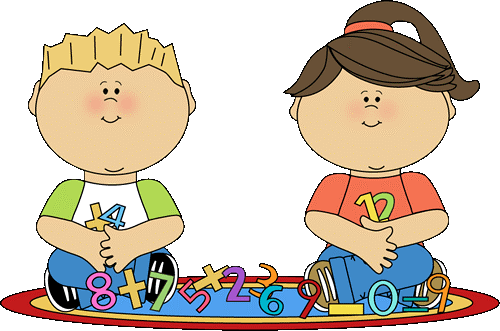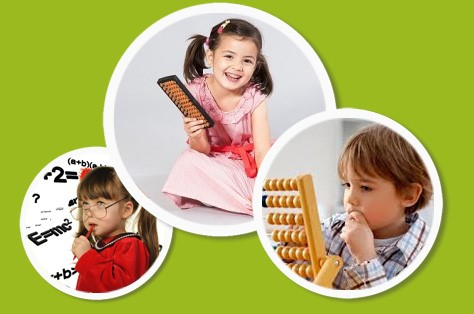Home » Ideas » Creativity » How to teach Mental Arithmetic for Kids# How to teach Mental Arithmetic for Kids

## Recently, there are a lot of ways to teach the math for children but the way to teach mental arithmetic is focusing more because its benefits bring.

If you spend time for learn more about the problem, you will know that there are lot of tips and way for training for kids how to use mental arithmetic on YouTube or Google. However, almost the ways are not interesting to those who start school. It is the reason why parents ask the question How to make her feel interesting with this. The answer is that you let’s take a look through the clip fast mental skills, article on the benefits of SWUN Math and the SWUN math app and tips clip lesson are extremely memorable which can make children will be interested and fascinated with the numbers. Furthermore, you can refer to the step for helping our kids learn the way to mental arithmetic.Step 1

First of all, you should help your child develop a strong sense of numbers and help children understand the meaning of numbers before understanding the concept of plus and minus. For example, you can ask the child how many different ways to make 6; children can answer pairs of 0 and 6, 5 and 1, 4 and 2, 3 and 3 and etc. This will help children generate real understanding and then abstract example. Moreover, the note for you is that you should take example with small number from 1 to 10 because the main purpose of the step is help children get acquainted with the numbers. Therefore, if your children do a wrong number, you should not angry with them.

Step 2

After getting acquainted with the numbers, you can teach counting how 2 units. For example, let’s count kids 2, 4, 6, 8, 10 and so on. Gather numbers that help children understand that adding will ignore the next number, except for the previous number. If you study up to 100 it is possible for your child to count 5, 10, 15 … 100. This step can help children can understand about the plus as well as how to count the number.

Step 3

This is common way with almost parent when teaching count for their children. You should use your fingers or other materials such as ballpoint pens, calculators, etc. to teach your child to add or subtract. After your children can plus and minus with number, you can raise the difficult of calculation by teach children how to imagine. For example, there are 3 horses in the cage, one running out for the rest of the imaginary kids. Counting by image will help children improve image ability with the number.

Step 4

In fact, when learning with numbers can make children feeling boring, so parents should combine the way for teaching with fun tricks to help children pay attention to plus and minus. For example, you can use quick example of addition, subtraction such as 3 + 3 =? Or 5 + 0 =?, 7 – 0 =? Or 100 + 0 =? Although children learn only in small numbers, it will make children easier when counting.Step 5

Furthermore, practical plus and minus in the usual way with cards, rods and etc. can make children also be bored. Therefore, you can search for software and programs on the Internet can also be exciting. In fact, if you spend time for research, there are a lot of quality and interesting app for learning math on phone such as the SWUN math app which is perfect for students to improve their all-round mathematics ability. If you can give children time to practice to motivate children and more exciting. Moreover, change the environment for learning is also the best way to improve the focusing and effective of learning. Therefore, you can learn math with children when walking, shopping or playing. For example, when walking on street, you can ask your children to find the any number on the street and using plus and minus with them. The way wills more interesting than learning from book or phone.

Besides these steps, you can read some examples below to know more about how to teach mental arithmetic for children.

Mental arithmetic with plus

• When adding two numbers, put large numbers in front of a small number and count them up in the beginning

For example, when doing 6 + 29, you should tell your children that he should put 29 + 6. So children will be easier to remember because only counting from 29.

• Plus from about 10 units at a time

For example, 55 + 24 would be = 55 + 10 + 10 + 4 = 55, 65, 75 + 4

• Split up for a dozen rounds and add up

Example: 37 + 15 = 37 + 3 + 12 = 40 + 12

38 + 37 = 40 + 40 – 2 – 3 = 75

• Use a round number near the dozen and then subtract the excess

Example: 35 + 18 = 35 + 20 – 2 = 55 – 2 = 53

• Break the numbers into rounds and then add up to odd individual

For example: 35 + 24 = 30 + 20 + 5 + 4 = 50 + 9 = 59

Mental arithmetic with minus

• Count counting from small numbers up to near ten

For example: 23 – 18 = counting from 18 to 20 is 2 units, from 20 to 23 is 3 units

• Split the numbers for a dozen and then divide

For example: 83 – 42 = 83 – 40 – 2 = 43 – 2 = 41

• Use nearby round numbers and add extra numbers:

For example: 43 – 19 = 43 – 20 + 1 = 23 + 1 = 24